Author: Oscar Cronquist Article last updated on January 26, 2023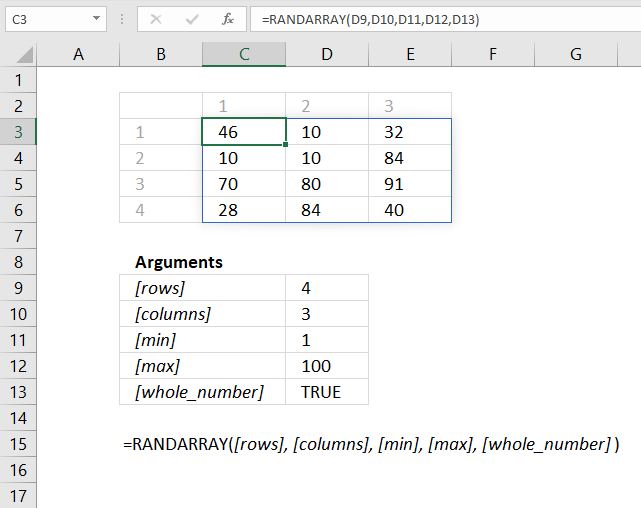The RANDARRAY function returns a table of random numbers across rows and columns. It is located in the Math and Trigonometry category and is only available to Excel 365 subscribers.

## 1. RANDARRAY Function Syntax

RANDARRAY([rows], [columns], [min], [max], [whole_number])

## 2. RANDARRAY Function Arguments

 Arguments Text [rows] Optional. Number of rows., 1 is the default value. [columns] Optional. Number of columns, 1 is the default value. [min] Optional. The smallest number. [max] Optional. The largest number. [whole_number] Optional. TRUE - whole number. FALSE - decimal number.

## 3. RANDARRAY Function exampleFormula in cell B3:

=RANDARRAY(D9,D10,D11,D12,D13)

The formula demonstrated in the image above returns a table of four rows and three columns populated with random numbers from 1 to 100. The formula returns new values every time you press F9.

## 4. What is a spilled array formula?

Excel 365 automatically expands the output range based on the number of values in the array, without requiring the user to enter the formula as an array formula.

This new behavior of Excel is called spilled array formula and is something only dynamic array formulas can do. Dynamic array formulas are only available to Excel 365 subscribers.

## 5. Why does the RANDARRAY function return a #SPILL! error?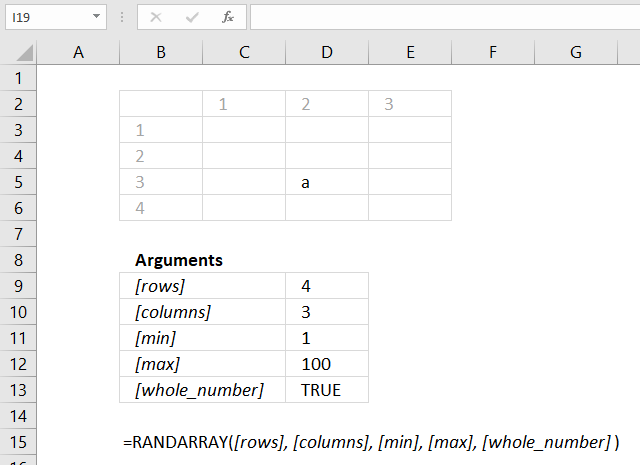#SPILL! error is returned by the RANDARRAY function if the required cell range is populated by any other value. You have two options:

• Remove value leaving the cell blank.
• Enter the dynamic formula in another cell that has empty adjacent cells.

## 6. Why does the RANDARRAY function return a #NAME! error?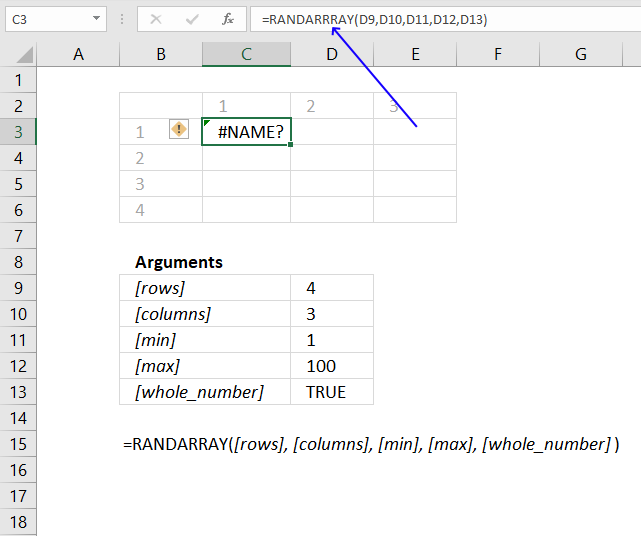If a cell returns #NAME! error you have either misspelled the function name or you are using an older incompatible Excel version.

The image above shows that I misspelled the RANDARRAY function in the formula bar, cell F3 displays #NAME! error.

Only Excel 365 subscription version supports the new dynamic array formula like the RANDARRAY function, older Excel versions like Excel 2019, 2016, 2013, 2010, 2007, and earlier versions do not support the RANDARRAY function.

Here is how to find out your Excel version: Get your Excel version

## 7. How to create a list of unique distinct numbers sorted in random order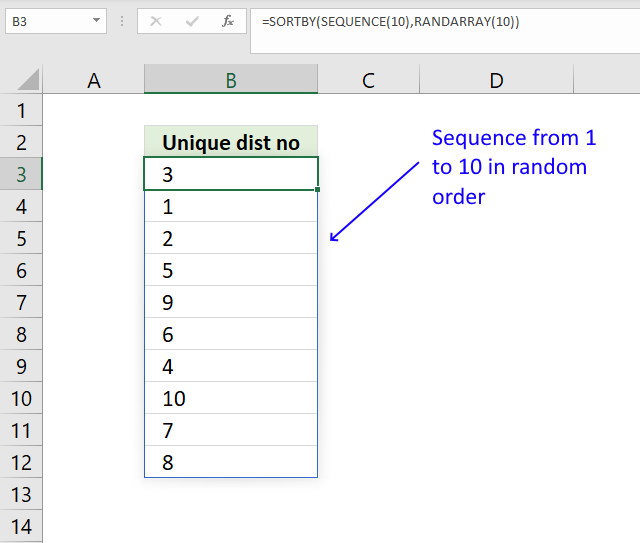The image above shows a formula in cell B3 that returns numbers from one to ten in random order.

Formula in cell D3:

=SORTBY(SEQUENCE(10),RANDARRAY(10))

### Explaining formula in cell D3

#### Step 1 - Create an array of random no between 0 (zero) and 1

RANDARRAY(10)

returns

{0.00631985584666483; 0.846522787602261; 0.817086790647678; 0.0907043159731944; 0.831202119264657; 0.218031433770172; 0.505530171355597; 0.80026556794421; 0.853884408869484; 0.487721931499081}

#### Step 2 - Create an array of numbers between 1 and 10

The SEQUENCE function returns a sequence of numbers. SEQUENCE(rows, [columns], [start], [step])

SEQUENCE(10)

returns

{1; 2; 3; 4; 5; 6; 7; 8; 9; 10}

#### Step 3 - Sort sequence based on random numbers

The SORTBY function sorts the sequence of numbers based on the array of random numbers. SORTBY(array, by_array1, [sort_order1], [by_array2, sort_order2],…)

SORTBY(SEQUENCE(10),RANDARRAY(10))

becomes

SORTBY({1; 2; 3; 4; 5; 6; 7; 8; 9; 10}, {0.00631985584666483; 0.846522787602261; 0.817086790647678; 0.0907043159731944; 0.831202119264657; 0.218031433770172; 0.505530171355597; 0.80026556794421; 0.853884408869484; 0.487721931499081})

and returns

{8; 5; 3; 7; 10; 9; 1; 2; 6; 4}.

## 8. How to shuffle characters in a text string randomly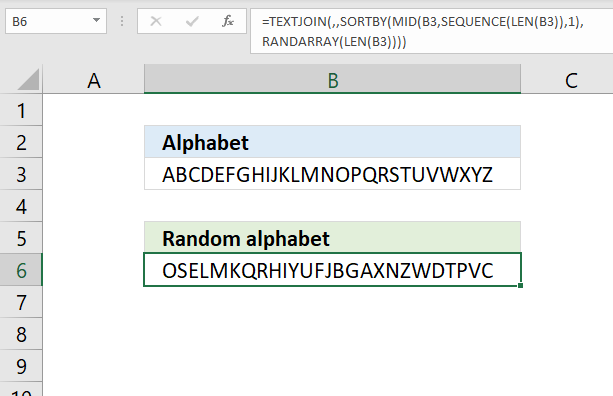The image above shows a formula in cell B6 that returns carachters in random order based on the original text string in cell B3.

Formula in cell D3:

=TEXTJOIN(, , SORTBY(MID(B3, SEQUENCE(LEN(B3)), 1), RANDARRAY(LEN(B3))))

### Explaining formula in cell D3

#### Step 1 - Count rows in cell range

The LEN function counts the number of characters in a string.

LEN(B3)

#### Step 2 - Create sequence based on the number of characters in B3

The SEQUENCE function returns a sequence of numbers. SEQUENCE(rows, [columns], [start], [step])

SEQUENCE(LEN(B3))

becomes

SEQUENCE(26)

and returns

{1; 2; 3; 4; 5; 6; 7; 8; 9; 10; 11; 12; 13; 14; 15; 16; 17; 18; 19; 20; 21; 22; 23; 24; 25; 26}

#### Step 3 - Split text string into characters

The MID function returns a substring from a string based on the starting position and the number of characters you want to extract. MID(text, start_num, num_chars)

MID(B3, SEQUENCE(LEN(B3)), 1)

becomes

MID("ABCDEFGHIJKLMNOPQRSTUVWXYZ", {1; 2; 3; 4; 5; 6; 7; 8; 9; 10; 11; 12; 13; 14; 15; 16; 17; 18; 19; 20; 21; 22; 23; 24; 25; 26}, 1)

and returns

{"A"; "B"; "C"; "D"; "E"; "F"; "G"; "H"; "I"; "J"; "K"; "L"; "M"; "N"; "O"; "P"; "Q"; "R"; "S"; "T"; "U"; "V"; "W"; "X"; "Y"; "Z"}

#### Step 4 - Create an array of random numbers

RANDARRAY(LEN(B3))

becomes

RANDARRAY(26)

and returns an array of 26 random decimal numbers.

#### Step 5 - Sort array of characters based on random numbers

The SORTBY function sorts the characters based on the array of random numbers. SORTBY(array, by_array1, [sort_order1], [by_array2, sort_order2],…)

SORTBY(MID(B3, SEQUENCE(LEN(B3)), 1), RANDARRAY(LEN(B3)))

becomes

SORTBY({"A"; "B"; "C"; "D"; "E"; "F"; "G"; "H"; "I"; "J"; "K"; "L"; "M"; "N"; "O"; "P"; "Q"; "R"; "S"; "T"; "U"; "V"; "W"; "X"; "Y"; "Z"}, RANDARRAY(LEN(B3)))

and returns

{"T"; "H"; "L"; "R"; "F"; "X"; "O"; "V"; "K"; "S"; "G"; "D"; "B"; "Q"; "U"; "J"; "P"; "C"; "I"; "E"; "Z"; "Y"; "A"; "W"; "M"; "N"}

#### Step 6 - Join characters in array

The TEXTJOIN function concatenates the characters and returns a text string.

TEXTJOIN(, , SORTBY(MID(B3, SEQUENCE(LEN(B3)), 1), RANDARRAY(LEN(B3))))

becomes

TEXTJOIN(, , {"T"; "H"; "L"; "R"; "F"; "X"; "O"; "V"; "K"; "S"; "G"; "D"; "B"; "Q"; "U"; "J"; "P"; "C"; "I"; "E"; "Z"; "Y"; "A"; "W"; "M"; "N"})

and returns THLRFXOVKSGDBQUJPCIEZYAWMN.

## 9. How to create a random text string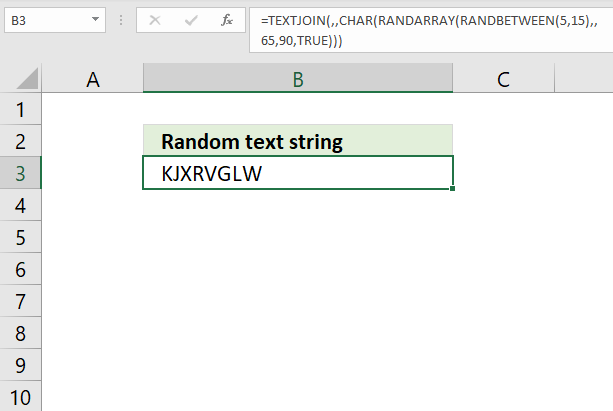The image above shows a formula that creates characters from A to Z with a total string length of 5 to 15 characters. Press F9 to recalculate the formula.

Formula in cell B6:

=TEXTJOIN(, , CHAR(RANDARRAY(RANDBETWEEN(5, 15), , 65, 90,TRUE)))

### Explaining formula in cell D3

#### Step 1 - Create a random number between 5 and 15

The RANDBETWEEN function returns a random whole number between the numbers you specify. RANDBETWEEN(bottomtop)

RANDBETWEEN(5, 15)

For some reason I don't know the RANDARRAY function can' be used in the first argument in another RANDARRAY function, in other words, a nested RANDARRAY function does not work.

#### Step 2 - Create an array of whole numbers between

The RANDARRAY function returns an array of whole numbers between 65 and 90. The size of the array is determined by the output from the RANDBETWEEN function.

RANDARRAY(RANDBETWEEN(5, 15), , 65, 90,TRUE)

returns

{89; 69; 73; 66; 72; 89; 72; 89; 70; 81; 85; 65; 76; 68}

#### Step 3 - Convert numbers to letters

The CHAR function converts a number to the corresponding character.

CHAR(RANDARRAY(RANDBETWEEN(5, 15), , 65, 90,TRUE))

becomes

CHAR({89; 69; 73; 66; 72; 89; 72; 89; 70; 81; 85; 65; 76; 68})

and returns

{"Y";"E";"I";"B";"H";"Y";"H";"Y";"F";"Q";"U";"A";"L";"D"}

#### Step 6 - Join characters in array

The TEXTJOIN function concatenates the characters and returns a text string.

TEXTJOIN(, , CHAR(RANDARRAY(RANDBETWEEN(5, 15), , 65, 90,TRUE)))

becomes

TEXTJOIN(, , {"Y";"E";"I";"B";"H";"Y";"H";"Y";"F";"Q";"U";"A";"L";"D"})

and returns YEIBHYHYFQUALD.

### Get the Excel fileSEQUENCE-function.xlsx